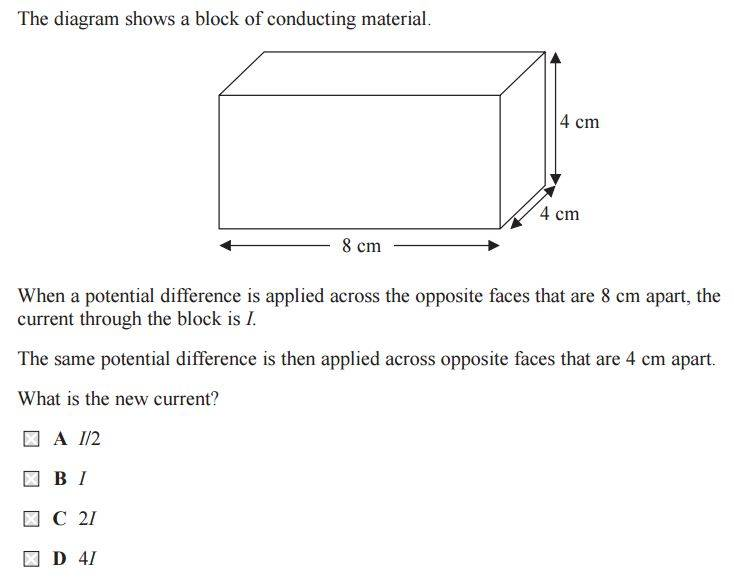# Current in a Wire

• ravsterphysics
In summary, the conversation discusses a scenario where a pd is applied across different faces of a rectangle and how it affects the current and resistance. The correct answer is D because both the distance the current has to travel and the area the current moves through change, resulting in a quarter of the original resistance. The relevant equation is R \propto \frac{l}{A} where l is the length of the resistor and A is the cross-sectional area.

## Homework Statement## The Attempt at a Solution

I know I = nqvA

When the pd is applied the surface that is 8cm long, the cross sectional area is 32cm (8x4) but when the pd is applied across the 4cm side, the cross sectional area is now 16cm (4x4) so I has decreased by a factor of 2 so the new I is I/2 thus answer A? But the correct answer is D.

When comparing I=nqvA for each case, you assumed that only A changed. q stays the saem OK - but is v the same?
HInt: Ohms' law and resistivity.

•ravsterphysics
You've got the order of the faces backwards. The initial scenario has the PD applied across the ends of the rectangle (the 4 x 4 ends are separated by 8 cm).

Also, remember that resistance is proportional to path length as well as being inversely proportional to the cross sectional area. Look up the definition of resistivity.

•ravsterphysics
ravsterphysics said:

## Homework Statement

View attachment 111643

## The Attempt at a Solution

I know I = nqvA

When the pd is applied the surface that is 8cm long, the cross sectional area is 32cm (8x4) but when the pd is applied across the 4cm side, the cross sectional area is now 16cm (4x4) so I has decreased by a factor of 2 so the new I is I/2 thus answer A? But the correct answer is D.

I think that the relevant equation is: $R \propto \frac{l}{A}$ where $l$ is the length of the resistor and $A$ is the cross-sectional area. Switching from one face to the other changes both $A$ and $l$.

•ravsterphysics
People are always very quick to use formulas ... much easier just to look at it ...the distance the current has to travel has halved so this alone will make the resistance halve ... but in addition the area the current moves through has doubled .. this will also halve the resistance ... so in total the resistance has dropped to a quarter of what it was ...

•ravsterphysics
Simon Bridge said:
When comparing I=nqvA for each case, you assumed that only A changed. q stays the saem OK - but is v the same?
HInt: Ohms' law and resistivity.

gneill said:
You've got the order of the faces backwards. The initial scenario has the PD applied across the ends of the rectangle (the 4 x 4 ends are separated by 8 cm).

Also, remember that resistance is proportional to path length as well as being inversely proportional to the cross sectional area. Look up the definition of resistivity.

stevendaryl said:
I think that the relevant equation is: $R \propto \frac{l}{A}$ where $l$ is the length of the resistor and $A$ is the cross-sectional area. Switching from one face to the other changes both $A$ and $l$.

oz93666 said:
People are always very quick to use formulas ... much easier just to look at it ...the distance the current has to travel has halved so this alone will make the resistance halve ... but in addition the area the current moves through has doubled .. this will also halve the resistance ... so in total the resistance has dropped to a quarter of what it was ...

Yep, I totally disregarded the effects on the wire's resistance. It's clear now why current goes up by a factor of 4. thanks for the help.

•Simon Bridge